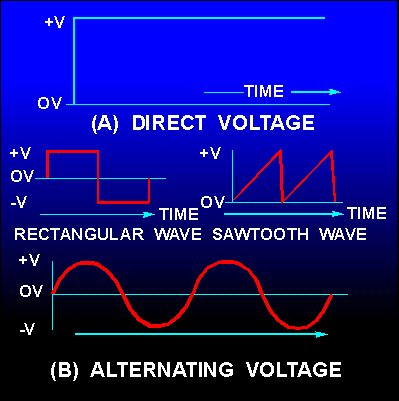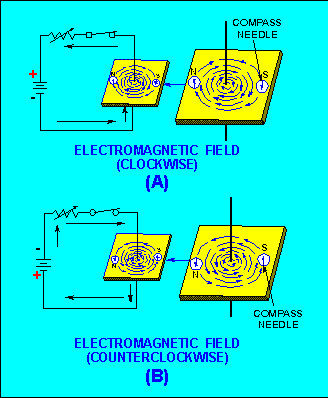voltage, that is, direct current and voltage and alternating current and voltage. If a graph is constructed showing the amplitude of a dc voltage across the terminals of a battery with respect to time, it will appear in figure 1-1 view A. The dc voltage is shown to have a constant amplitude.">Custom SearchVOLTAGE WAVEFORMS You now know that there are two types of current and voltage, that is, direct current and voltage and alternating current and voltage. If a graph is constructed showing the amplitude of a dc voltage across the terminals of a battery with respect to time, it will appear in figure 1-1 view A. The dc voltage is shown to have a constant amplitude. Some voltages go through periodic changes in amplitude like those shown in figure 1-1 view B. The pattern which results when these changes in amplitude with respect to time are plotted on graph paper is known as a WAVEFORM. Figure 1-1 view B shows some of the common electrical waveforms. Of those illustrated, the sine wave will be dealt with most often. Figure 1-1. - Voltage waveforms: (A). Direct voltage; (B) Alternating voltage.ELECTROMAGNETISM The sine wave illustrated in figure 1-1 view B is a plot of a current which changes amplitude and direction. Although there are several ways of producing this current, the method based on the principles of electromagnetic induction is by far the easiest and most common method in use. The fundamental theories concerning simple magnets and magnetism were discussed in Module 1, but how magnetism can be used to produce electricity was only briefly mentioned. This module will give you a more in-depth study of magnetism. The main points that will be explained are how magnetism is affected by an electric current and, conversely, how electricity is affected by magnetism. This general subject area is most often referred to as ELECTROMAGNETISM. To properly understand electricity you must first become familiar with the relationships between magnetism and electricity. For example, you must know that: An electric current always produces some form of magnetism. The most commonly used means for producing or using electricity involves magnetism. The peculiar behavior of electricity under certain conditions is caused by magnetic influences. In 1819 Hans Christian Oersted, a Danish physicist, found that a definite relationship exists between magnetism and electricity. He discovered that an electric current is always accompanied by certain magnetic effects and that these effects obey definite laws. MAGNETIC FIELD AROUND A CURRENT-CARRYING CONDUCTOR If a compass is placed in the vicinity of a current-carrying conductor, the compass needle will align itself at right angles to the conductor, thus indicating the presence of a magnetic force. You can demonstrate the presence of this force by using the arrangement illustrated in figure 1-2. In both (A) and (B) of the figure, current flows in a vertical conductor through a horizontal piece of cardboard. You can determine the direction of the magnetic force produced by the current by placing a compass at various points on the cardboard and noting the compass needle deflection. The direction of the magnetic force is assumed to be the direction in which the north pole of the compass points. Figure 1-2. - Magnetic field around a current-carrying conductor.In figure 1-2 (A), the needle deflections show that a magnetic field exists in circular form around the conductor. When the current flows upward (see figure 1-2(A)), the direction of the field is clockwise, as viewed from the top. However, if you reverse the polarity of the battery so that the current flows downward (see figure 1-2(B)), the direction of the field is counterclockwise. The relation between the direction of the magnetic lines of force around a conductor and the direction of electron current flow in the conductor may be determined by means of the LEFT-HAND RULE FOR A CONDUCTOR: if you grasp the conductor in your left hand with the thumb extended in the direction of the electron flow (current) (- to +), your fingers will point in the direction of the magnetic lines of force. Now apply this rule to figure 1-2. Note that your fingers point in the direction that the north pole of the compass points when it is placed in the magnetic field surrounding the wire. An arrow is generally used in electrical diagrams to denote the direction of current in a length of wire (see figure 1-3(A)).Where a cross section of a wire is shown, an end view of the arrow is used. A cross-sectional view of a conductor that is carrying current toward the observer is illustrated in figure 1-3(B). Notice that the direction of current is indicated by a dot, representing the head of the arrow. A conductor that is carrying current away from the observer is illustrated in figure 1-3(C). Note that the direction of current is indicated by a cross, representing the tail of the arrow. Also note that the magnetic field around a current-carrying conductor is perpendicular to the conductor, and that the magnetic lines of force are equal along all parts of the conductor. Figure 1 - 3. - Magnetic field around a current-carrying conductor, detailed view.When two adjacent parallel conductors are carrying current in the same direction, the magnetic lines of force combine and increase the strength of the field around the conductors, as shown in figure 1-4(A).Two parallel conductors carrying currents in opposite directions are shown in figure 1-4(B). Note that the field around one conductor is opposite in direction to the field around the other conductor. The resulting lines of force oppose each other in the space between the wires, thus deforming the field around each conductor. This means that if two parallel and adjacent conductors are carrying currents in the same direction, the fields about the two conductors aid each other. Conversely, if the two conductors are carrying currents in opposite directions, the fields about the conductors repel each other. Figure 1-4. - Magnetic field around two parallel conductors.Q.6 When placed in the vicinity of a current-carrying conductor, the needle of a compass becomes aligned at what angle to the conductor? Q.7 What is the direction of the magnetic field around a vertical conductor when (a) the current flows upward and (b) the current flows downward? Q.8 The "left-hand rule" for a conductor is used for what purpose? Q.9 In what direction will the compass needle point when the compass is placed in the magnetic field surrounding a wire? Q.10 When two adjacent parallel wires carry current in the same direction, the magnetic field about one wire has what effect on the magnetic field about the other conductor? Q.11 When two adjacent parallel conductors carry current in opposite directions, the magnetic field about one conductor has what effect on the magnetic field about the other conductor?Integrated Publishing, Inc. - A (SDVOSB) Service Disabled Veteran Owned Small Business